# mne.time_frequency.psd_welch#

mne.time_frequency.psd_welch(inst, fmin=0, fmax=inf, tmin=None, tmax=None, n_fft=256, n_overlap=0, n_per_seg=None, picks=None, proj=False, n_jobs=None, reject_by_annotation=True, average='mean', window='hamming', *, verbose=None)[source]#

Compute the power spectral density (PSD) using Welch’s method.

Calculates periodograms for a sliding window over the time dimension, then averages them together for each channel/epoch.

Parameters
instinstance of `Epochs` or `Raw` or `Evoked`

The data for PSD calculation.

fmin`float`

Min frequency of interest.

fmax`float`

Max frequency of interest.

tmin

Min time of interest.

tmax

Max time of interest.

n_fft`int`

The length of FFT used, must be `>= n_per_seg` (default: 256). The segments will be zero-padded if `n_fft > n_per_seg`. If n_per_seg is None, n_fft must be <= number of time points in the data.

n_overlap`int`

The number of points of overlap between segments. Will be adjusted to be <= n_per_seg. The default value is 0.

n_per_seg

Length of each Welch segment (windowed with a Hamming window). Defaults to None, which sets n_per_seg equal to n_fft.

picks

Channels to include. Slices and lists of integers will be interpreted as channel indices. In lists, channel type strings (e.g., ```['meg', 'eeg']```) will pick channels of those types, channel name strings (e.g., `['MEG0111', 'MEG2623']` will pick the given channels. Can also be the string values “all” to pick all channels, or “data” to pick data channels. None (default) will pick good data channels (excluding reference MEG channels). Note that channels in `info['bads']` will be included if their names or indices are explicitly provided.

projbool

Apply SSP projection vectors. If inst is ndarray this is not used.

n_jobs

The number of jobs to run in parallel. If `-1`, it is set to the number of CPU cores. Requires the `joblib` package. `None` (default) is a marker for ‘unset’ that will be interpreted as `n_jobs=1` (sequential execution) unless the call is performed under a `joblib.parallel_backend()` context manager that sets another value for `n_jobs`.

reject_by_annotationbool

Whether to omit bad segments from the data before fitting. If `True` (default), annotated segments whose description begins with `'bad'` are omitted. If `False`, no rejection based on annotations is performed.

Has no effect if `inst` is not a `mne.io.Raw` object.

New in version 0.15.0.

average

How to average the segments. If `mean` (default), calculate the arithmetic mean. If `median`, calculate the median, corrected for its bias relative to the mean. If `None`, returns the unaggregated segments.

New in version 0.19.0.

window

Windowing function to use. See `scipy.signal.get_window()`.

New in version 0.22.0.

verbose

Control verbosity of the logging output. If `None`, use the default verbosity level. See the logging documentation and `mne.verbose()` for details. Should only be passed as a keyword argument.

Returns
psds`ndarray`, shape (…, n_freqs) or (…, n_freqs, n_segments)

The power spectral densities. If `average='mean` or `average='median'` and input is of type Raw or Evoked, then psds will be of shape (n_channels, n_freqs); if input is of type Epochs, then psds will be of shape (n_epochs, n_channels, n_freqs). If `average=None`, the returned array will have an additional dimension corresponding to the unaggregated segments.

freqs`ndarray`, shape (n_freqs,)

The frequencies.

Notes

New in version 0.12.0.

## Examples using `mne.time_frequency.psd_welch`#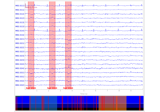Rejecting bad data spans and breaks

Rejecting bad data spans and breaks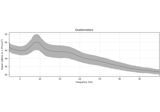Frequency and time-frequency sensor analysis

Frequency and time-frequency sensor analysis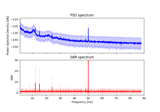Frequency-tagging: Basic analysis of an SSVEP/vSSR dataset

Frequency-tagging: Basic analysis of an SSVEP/vSSR dataset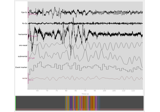Sleep stage classification from polysomnography (PSG) data

Sleep stage classification from polysomnography (PSG) data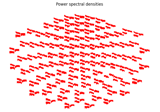Plot custom topographies for MEG sensors

Plot custom topographies for MEG sensors